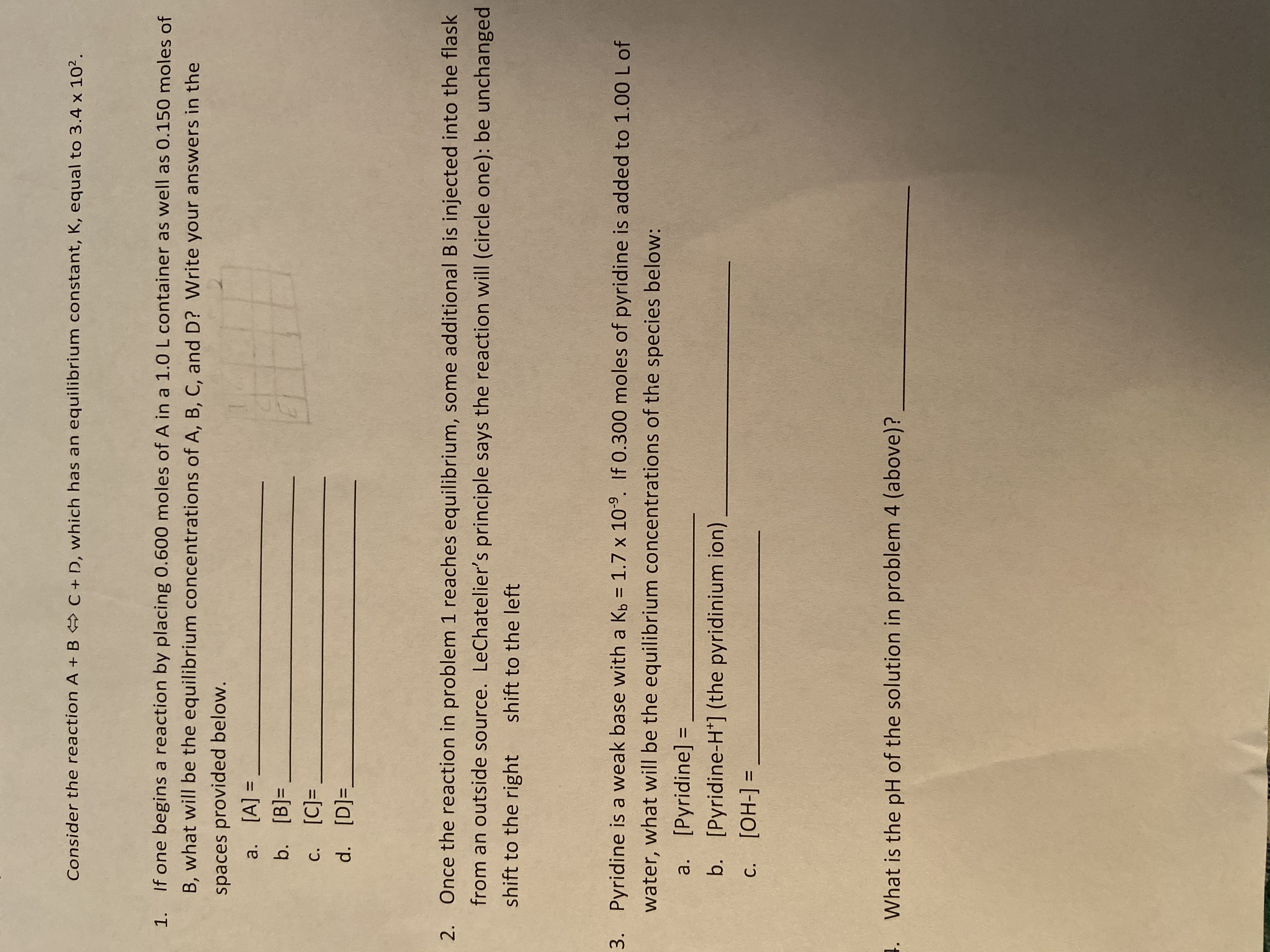# Consider the reaction A + B C+D, which has an equilibrium constant, K, equal to 3.4 x 102. If one begins a reaction by placing 0.600 moles of A in a 1.0 L container as well as 0.150 moles of 1. B, what will be the equilibrium concentrations of A, B, C, and D? Write your answers in the spaces provided below. a. [А] b. [B]= [C]= С. d. [D]= Once the reaction in problem 1 reaches equilibrium, some additional B is injected into the flask 2. from an outside source. LeChatelier's principle says the reaction will (circle one): be unchanged shift to the right shift to the left Pyridine is a weak base with a Kb 1.7 x 109. If 0.300 moles of pyridine is added to 1.00 L of 3. water, what will be the equilibrium concentrations of the species below: a. [Pyridine] = b. [Pyridine-H] (the pyridinium ion) [ОН-] 3 С. What is the pH of the solution in problem 4 (above)? .

Questionhelp_outlineImage TranscriptioncloseConsider the reaction A + B C+D, which has an equilibrium constant, K, equal to 3.4 x 102. If one begins a reaction by placing 0.600 moles of A in a 1.0 L container as well as 0.150 moles of 1. B, what will be the equilibrium concentrations of A, B, C, and D? Write your answers in the spaces provided below. a. [А] b. [B]= [C]= С. d. [D]= Once the reaction in problem 1 reaches equilibrium, some additional B is injected into the flask 2. from an outside source. LeChatelier's principle says the reaction will (circle one): be unchanged shift to the right shift to the left Pyridine is a weak base with a Kb 1.7 x 109. If 0.300 moles of pyridine is added to 1.00 L of 3. water, what will be the equilibrium concentrations of the species below: a. [Pyridine] = b. [Pyridine-H] (the pyridinium ion) [ОН-] 3 С. What is the pH of the solution in problem 4 (above)? . fullscreen

1 Rating

### Want to see this answer and more?

Experts are waiting 24/7 to provide step-by-step solutions in as fast as 30 minutes!*